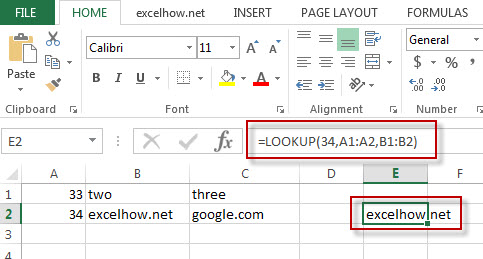# Excel Lookup Function

This post will guide you how to use Excel LOOKUP function with syntax and examples in Microsoft excel.

## Description

The Excel LOOKUP function will search a value in a vector or array.

The LOOKUP function is a build-in function in Microsoft Excel and it is categorized as a Lookup and Reference Function.

The LOOKUP function is available in Excel 2016, Excel 2013, Excel 2010, Excel 2007, Excel 2003, Excel XP, Excel 2000, Excel 2011 for Mac.

## Syntax

The syntax of the LOOKUP function is as below:

`= LOOKUP (lookup_value, lookup_vector, [result_vector])`

Where the LOOKUP function arguments are:
Lookup_value -This is a required argument.  A value that you want to search in the lookup_vector.
Lookup_vector – This is a required argument.  A excel Range that only contains one row or one column.
Result_vector – This is an optional argument. A excel Range that only contains one row or one column. It must be the same size as Lookup_vector. The lookup function will look up the value in the lookup_value range and returns the value from the same position in the result_vector.
Note: if Result_vector is omitted, the Lookup function will return the first column data.

## Example

The below examples will show you how to use Excel LOOKUP Lookup and Reference Function to search a value from a vector or array.

#1 To look up the value “34”in  A1:A2 range, then get the value from the same row or column in B1:B2 range, just using the following excel formula: =LOOKUP(“34”,A1:A2,B1:B2)### More Excel LOOKUP Formula Examples

• Finding the Max and Min value in an Alphanumeric Data
if you want to find the Maximal or minimal string value from an alphanumeric data list in the range B1:B5, you can create a formula based on the LOOKUP function and the COUNTIF function.….
• Convert Weekday Name to Number
Assuming that you have list of data B1:B7 that contain weekday names, you want to convert all weekday names to numbers.You can use a formula based on the MATCH function to achieve the result
• List all Worksheet Names
Assuming that you have a workbook that has hundreds of worksheets and you want to get a list of all the worksheet names in the current workbook. And the below will introduce 3 methods with you..…
• Copy and Paste Only Non-blank Cells
If you want only copy non-blank cells in a range in Excel, you need to select the non-blank cells firstly, then press Ctrl +C keys to copy the selected cells. So how to only select all non-blank cells in the selected range in your worksheet..…